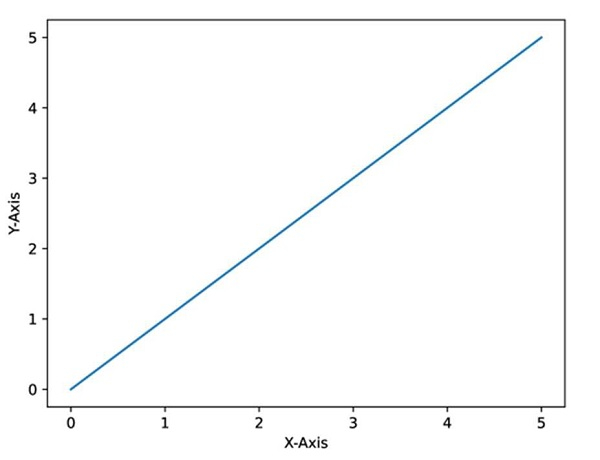# Saving images in Python at a very high quality

To save the images in Python with very high quality, you need to follow the steps given below −

• Create fig and ax variables using subplots method, where default nrows and ncols are 1.

• Plot the lines using plot() method.

• We can add axes labels using ylabel() and xlabel().

• To get a high-quality image, we can use .eps image format.

• You can increase the dot per inch value, i.e., dpi.

• Using savefig() method, we can save the image locally.

• To show the figure, use plt.show().

## Example

import matplotlib.pyplot as plt

fig, ax = plt.subplots()

plt.plot([0, 5], [0, 5])

plt.ylabel("Y-axis ")
plt.xlabel("X-axis ")

image_format = 'eps' # e.g .png, .svg, etc.
image_name = 'myimage.eps'

fig.savefig(image_name, format=image_format, dpi=1200)

## Output